Up | Logic - 1 | Blob1 - 61 | Intensity - 75 | PythonScript - 3 | BaseTool - 163 | SimpleMath - 21 | DummyTool - 20 | Goto - 59 | ColorMatcher - 54 | ImageCombiner - 74 | ImageFilter - 77 | ImageConverter - 55 | BarCodeReader - 56 | EasyBarCode - 72 | EasyMatrixCode - 73 | CommitImage - 159 | Separator - 102 Release Notes | Sample Profiles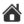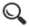SimpleMath - 21
 Tool Name: SimpleMathTool Tool Index: 21 Tool Category: Basic Tools

Description:

The SimpleMathTool tool performs the configured mathematical operations on the 2 input values.

The SimpleMathTool tool provides several mathematical functions which can be used to process the 2 inputs values to calculate the result value.
The input value can be a runtime value of any of any of the exposed parameters from the other tools configured in the Scorpion or can be a fixed value.

The SimpleMathTool tool processes in the 2 input values in three steps -
1. First step, which is the basic step, where the configured mathematical operation is done on the 2 input values
2. Second step, which is the 'Post processing Step 1', where the configured mathematical operation is done on the result from the basic step
3. Third step, which is the 'Post processing Step 2', where the configured mathematical operation is done on the result from 'Post processing Step 1'. The result from this third step is the final result from the SimpleMathTool  tool

User Interface:

(A) Tool Configuration dialog box - Setup Tab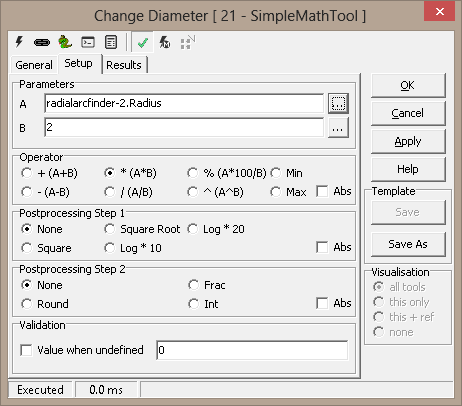1. The ‘Parameters’ group is used to configure the input values.
2. The 'A' textbox is used to specify the input value 1.
3. The 'B' textbox is used to specify the input value 2.
4. It is possible to type in fixed values for the ‘Parameters’ – ‘A’ and ‘B’. Also it is possible, to specify dynamic values for each one of these properties, by assigning them to any of the properties exposed from other tools defined in the Scorpion profile. The ‘…’ button available next to each of the ‘Parameters’ properties user elements can be clicked to select a tool and its parameter to be assigned.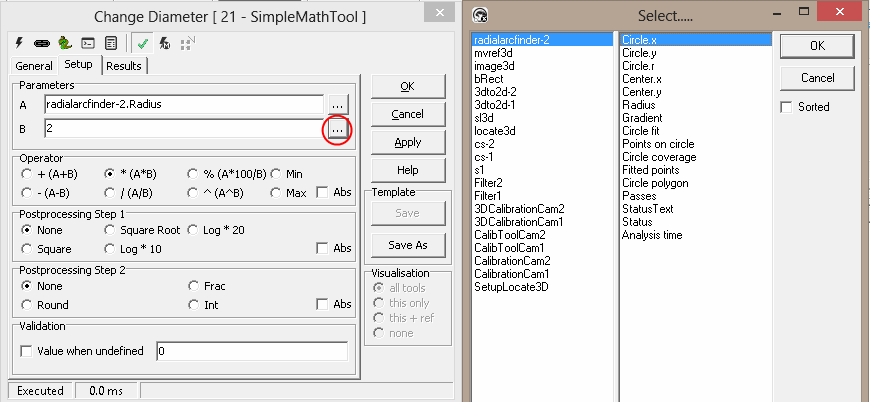5. The 'Operator' group is used for configuring the basic mathematical operation on the 2 input values. One of the following mathematical operations can be selected -
(a) + , addition of the 2 input values
(b) * , multiplication of the 2 input values
(c) % , percentage calculation of value A with respect to value B
(d) Min , finds minimum of the 2 input values and sets it as the result
(e) - , subtracting value B from value A
(f) / , division of value A by value B
(g) ^ , power, calculates A raised to B
(h) Max, finds maximum of the 2 input values and sets it as the result
6. If 'Abs' check-box is checked, it applies absolute value processing on the result calculated from the basic operation
7. The 'Postprocessing Step 1' is used for configuring the mathematical operation on the result value from the basic step. One of the following mathematical operations can be selected -
(a) None , no processing and the result is unchanged
(b) Square Root , calculates the square root value
(c) Log * 20 , calculates the logarithmic value and multiplies by 20
(d) Square , calculates the square value
(e) Log * 10 , calculates the logarithmic value and multiplies by 10
8. If 'Abs' check-box is checked, it applies absolute value processing on the result calculated from the post processing step 1
9. The 'Postprocessing Step 2' is used for configuring the mathematical operation on the result value from the post processing step 1. One of the following mathematical operations can be selected -
(a) None , no processing and the result is unchanged
(b) Frac , calculates the fraction value
(c) Round , calculates the rounded integer value
(d) Int , calculates the integer value
10. If 'Abs' check-box is checked, it applies absolute value processing on the result calculated from the post processing step 2, which is the final result from the processing
11. The 'Value when undefined' check-box under the 'Validation' group can be checked to apply validation on the final result. This can be checked to validate the final result, and setting the result value to a fixed configured value, if the final result is 'undefined'. The final result can be undefined, if any of the input values are undefined or the mathematical processing generates undefined result, eg division by zero.

Basic Processing when the tool is executed:

1. The 2 input values are received. Runtime values are obtained of any of the 2 input values are dynamic values.
2. Basic mathematical operation is done on the 2 input values, as per the operation configured in the 'Operator' group.
3. Mathematical operation, as configured in the 'Postprocessing Step 1' is done on the result from the basic mathematical operation.
4. Mathematical operation, as configured in the 'Postprocessing Step 2' is done on the result from the Postprocessing Step 1.
5. If enabled, the result from the 'Postprocessing Step 2' is validated to calculate the final result.

 Inputs to the Tool: Inputs: 1. Values of tool parameters used in the configuration, at the time of processing, if dynamic values are used for any of the configuration parameters Uses Reference: No Uses Image: No

Results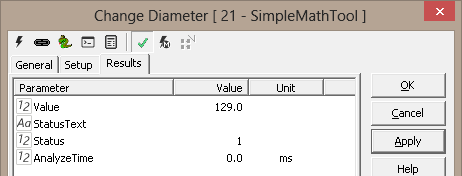Outputs from the Tool:
Outputs:
 1 Value: Numeric Final result from the SimpleMathTool, as per the configuration 2 StatusText: Text This is a standard output from all Scorpion tools and describes the processing status. 3 Status: Numeric This is a standard output from all Scorpion tools and indicates error/success of the tool processing. 1 indicates success and 0 indicates error. 4 AnalyzeTime: Numeric This is a standard output from all Scorpion tools and indicates the time taken by the last processing operation of this tool
Visualizations: None
Reference outputs: None

 Templates: Supports Templates: No

 Scorpion Vision Version XII : Build 646 - Date: 20170225 Scorpion Vision Software® is a registered trademark of Tordivel AS. Copyright © 2000 - 2017 Tordivel AS.